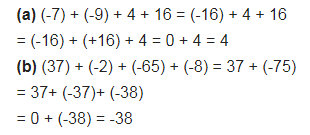# Find the sum: (a) (-7) + (-9) + 4 + 16

Find the sum:
(a) (-7) + (-9) + 4 + 16
(b) (37) + (-2) + (-65) + (-8)